# Maxwell's Equations

Click here to go to our page on finite integration technique (new for February 2015!)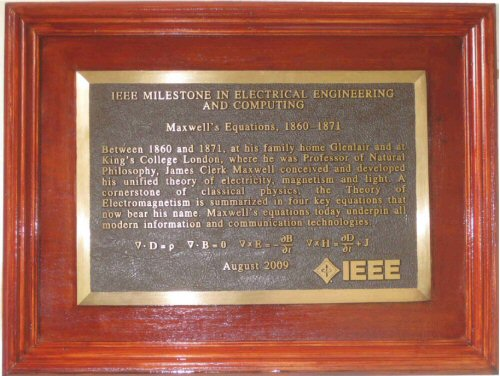IEEE Milestone, honoring James Clerk Maxwell and Maxwell's Equations, the equations bearing his name, unveiled on August 13, 2009 at Glenair House, Maxwell's family home in Kirkcudbrightshire, Scotland.

Dr. Rautio is the founder of Sonnet Software and has spent considerable personal time and money honoring Maxwell and restoring the Maxwell house (not the coffee that used to be manufactured in Hoboken). Read about Dr. Rautio here. Below is a series of videos of him talking about Maxwell's history, which is incomplete.  Hopefully, someday the MTT-S will pay a few bucks and have a professional video produced of this awesome lecture. How about we shoot it at the 2015 IMS in Phoenix?

Maxwell1

Maxwell2

Maxwell3

Maxwell4 Bagpipes and tigers!

Maxwell5

Maxwell6 Bullies and vampires

Maxwell7

Maxwell8

Maxwell9

Maxwell10

Maxwell11

Maxwell12

Maxwell13 death of Maxwell

Below, Dr. James Rautio explains Maxwell's equations (new for October 2014).

# Maxwell’s Equations

If you are up to a technical description of Maxwell’s equations and you are familiar with vector calculus, check out http://en.wikipedia.org/wiki/Maxwell%27s_equations. Rather than try to crudely duplicate that first rate and detailed description, here we present a much more physical and intuitive understanding. It is good if you understand that an integral is a sum of something or other, somehow, but even that is not really required.

First, here are the equations. Don’t let them scare you. As we go along, each term of each equation will start to feel like a friend. Then, when and if you learn some vector calculus, you will know the big picture up front. You will know where it is all going. You will understand.

1)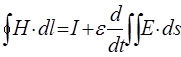2)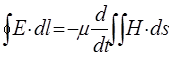3)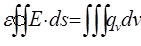4)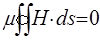In the late 1800’s, physics began a transition from playing with magnets to Einstein’s theory of relativity. This was brought about by what we now call “Maxwell’s Equations”. Maxwell (1831-1879) is the 19th century’s most famous physicist. Maxwell’s four equations, shown here in one of their modern forms, were actually discovered by several physicists. Maxwell realized that all the equations worked together and he added one term, something called “displacement current”. The set of equations is now called “Maxwell’s Equations” basically because he showed how all four worked together to completely define the field of “electromagnetics”. Although this may sound simple today, with what was known at that time, it was a true stroke of genius.

We call Maxwell’s equations “first principles” because they were not derived from other equations or theories. Rather, 19th century physicists did experiments with magnets, batteries, and such. Then, by trial and error, they developed mathematical equations which give the same answer as their experiments.

Maxwell’s four equations describe how magnetic fields and electric fields behave. For example, if you wrap a wire around a nail and connect a battery, you make a magnet. Maxwell’s first equation, Ampère’s Law tells us how the magnet will push or pull other magnets. According to the second equation, Faraday’s Law, you can take a magnet and spin it around to make an electric generator. This is where the electricity running your computer comes from. The third equation is Gauss’s law. This says static electricity (electric charge) generates voltage. You can see this on any dry day by shuffling across a rug and touching a door-knob. The fourth equation says that magnetic charge does not exist, a great mystery that physicists are still trying to understand.

Maxwell’s “displacement current” is the last term of the first equation. This has to do with what happens to electric fields when they change, for example when the static electricity from your finger arcs to the door knob. The electric field around your finger suddenly drops to zero. This sudden change the electric field generate a magnetic field. The second equation says the reverse is true. If you change a magnetic field, (say, by turning an electromagnet on and off) you generate an electric field. In fact, electric and magnetic fields can keep working together, all by themselves, each constantly changing, sometimes for millions of years. The result is what we know as light, or radio waves, or gamma rays, or, in general, “electromagnetic radiation”. If you want to see some million year old electromagnetic radiation, just look up into the sky some starry night.

At the end of the 19th century, a young Swiss patent clerk, Albert Einstein, learned about Maxwell’s equations and wondered what happens if a source of electromagnetic radiation (like a light bulb) were moving and he stood still. Then, what would happen if he were moving and the source stood still. If the movement speed approaches the speed of light, some really strange things happen. But, more importantly, he found that he could develop his theory assuming everything depended only on relative movement. Which one was actually moving and which one actually stood still was of absolutely no concern. Thus was born Einstein’s Theory of Relativity.

Even today, physicists can spend an entire life-time finding new solutions to Maxwell’s equations. But we sometimes lose sight of the fact that Maxwell’s equations do not explain why, for example, two magnets can attract or repel. They only allow us to calculate how strongly and in what direction the magnets are pulled. The question, “Why?” has yet to be answered.

# Magnetic Fields

First, wind a wire around a nail and connect a battery. We have a magnet.  However, when we connect the battery (assuming it is not a really big battery and the whole thing goes up in smoke!), nothing changes. We don’t see anything, we don’t feel anything. It looks just the same as it did before we connected the battery.

But we know that there is a magnetic field around the magnet. We know that the magnetic field can cause other magnets to be attracted or repelled. We know that the magnetic field is stronger close to the poles of the magnet and that a stronger magnetic field means the other magnets get pushed or pulled more strongly.  We know that whether the magnet gets pushed or pulled depends on where we put the magnets and at in what direction we hold the magnet. If we turn the magnet end-for-end, we can change a push into a pull. This is all caused by the magnetic field around the magnet. So what does the magnetic field look like? Let’s use the old iron filing trick:

Take a piece of paper with some iron filings on it. Put the paper over the magnet and shake it around. The iron filings form into lines. We call these “Magnetic Lines of Force”. By looking at the lines of force, we can see where the magnetic field is stronger. It is stronger when the lines are closer to each other. You can also see the direction of the magnetic field. The magnetic field has the same direction as the lines of force. If you put a piece of iron in the magnetic field, it will try to move along the lines of force to where the magnetic field is the strongest. This is why both the direction and the strength of the magnetic field is important.

Magnetic fields are used in making old fashioned (CRT, Cathode Ray Tube) television sets. There is a beam of electrons that starts in the back of the picture tube. Electromagnets along the side of the picture tube guide the beam to the front of the tube where the picture is made. The beam follows the lines of force set up by the magnets. The TV designer controls the electromagnets with high speed electronics so that the beam sweeps back and forth on the front of the picture tube, painting a picture as it goes.

The mathematical symbol for magnetic field is “H”. You can actually get meters that measure magnetic field. If the meter indicates a stronger field, then there will be more magnetic attraction or repulsion. A simple compass can be used to see the direction of the magnetic field. The earth’s magnetic field goes from south to north.

Now, for a surprise. Even though you can see the lines of force right there in those iron filings, they are not real. The reason you can see “lines of force” in the iron filings is because each little iron filing has become a magnet. So one small speck of iron filing-magnet attracts a second. This makes a stronger magnet. This stronger magnet attracts another iron filing.  And so it goes, until all the iron filings have formed into lines. The lines of iron filings just happen to form up depending on the magnetic field.

Faraday introduced the concept of lines of force. He found them useful for getting a practical understanding of what is going on. So even though lines of force are not real, they are still useful for the same purpose that Faraday intended. Don’t hesitate to imagine them when you try to understand what is happening when you make a magnet or build a television set.

# Electric Field

In our discussion of magnetic field, we point out that a field has two important quantities at every point, a direction and a strength. The same is true for electric field. Also, as with magnetic field, it is easy to visualize electric lines of force which help us see what an electric field is like.

Let’s start with another classic experiment. Find a Van de Graff generator and someone who has long fine hair and is very trusting. The Van de Graff generator makes static electricity. Start the generator and have the person put one hand on top of the generator. The person’s hair all stands on end (it works better on dry days, otherwise the static electricity leaks off into the air).

After laughing at the really strange sight, take a look at the hair. By touching the generator, static electricity flows from the generator to the person’s head. The person’s head is now full of static electricity. The static electricity generates an electric field around it. The electric field is what makes the person’s hair radiate out in all directions.

Look closely at the person’s hair. It makes sense that the electric field is strongest closest to the person’s head, where all the static electricity is. This is also where the person’s hair is closest together. Lines of force (the hair) being close together mean strong fields.

The hair also shows the direction of the electric field. All the hair radiates straight out from the person’s head. So do the lines of force. If you put something into an electric field, like hair, it will try to move along the lines of force. How much it gets pushed or pulled depends on the strength of the electric field.

You can get meters that measure electric field. Be careful, however. If the reading gets too strong, there will be arcs and sparks! This is what happens when you get zapped by touching a door knob or get struck by lightening.

Also, just like magnetic lines of force, electric lines of force are not real. But, imagining lines of force is very helpful when you need to visualize just exactly what an electric field looks like, especially on a bad hair day or if you get caught in a thunderstorm.

# Ampère’s LawThe first of Maxwell’s four equation is called Ampère’s Law. It has to do with an electric current creating a magnetic field. Anyone who has wrapped a wire around a nail and connected a battery has experienced Ampère’s Law. In fact electric current is today measured in “Amperes”, or “Amps” for short.

Ampère developed this equation by experimenting with magnets. If you pass an electric current through a wire, perhaps wound around an iron core (like a nail), you create a magnetic field.

Ampère found that sometimes a magnet would attract another magnet, but other times it would repel. Then he found that attraction or repulsion depends on the direction that current flows in the wire and in what orientation you hold the magnets. By trying different experiments and different equations, he gradually found that this equation (without the last term) could be used to calculate the results of his experiments.

This equation has to do with magnetic field, “H”, and current, “I”. To use this equation, you must first pick a closed curve. It can be any curve at all, anywhere you want. The only important restriction is that it be closed. A circle is a good example (notice the little circle in the very first symbol).

The left side of the equation says to first calculate the magnetic field along the entire length of the curve that you picked. Next, you must sum up all the magnetic field that is parallel to the curve. Magnetic field which is perpendicular to the curve is excluded.

The right side of the equation (ignoring the complicated looking last term) is the total current flowing through the same curve that we used for the left hand side.

What this equation says is that if we increase the current flowing through a closed curve, the total magnetic field around that curve also increases. If you care to actually calculate numbers, you can add up the magnetic field around the curve and tell exactly how much current is flowing through the curve (ignoring that pesky last term). There are actually meters that clamp around a wire and sum up the magnetic field. The meter then reads out how much current is flowing in the wire.

What is amazing is that we can pick any curve we want, anywhere, any shape, any time. As long as it is a closed curve, this equation works perfectly. Pretty nifty!

In practical terms, this law says that if you want a stronger magnet, put another battery on that coil of wire. Just don’t put too many batteries on it or it will go up in smoke and the magnetic field will be gone. But even if that happens, Ampère’s Law still works, anytime, anywhere, and with any closed curve.

Before we get to the very last complicated looking term, we shall explore the rest of Maxwell’s Equations.Faraday’s law, the second of Maxwell’s four equations, describes how a changing magnetic field produces an electric field. This is almost an exact mirror image of Ampère’s Law. Here “E” represents the electric field and “H” represents the magnetic field. Faraday first described this law in practical terms, then his good friend, Maxwell, translated the idea into this equation.

The left hand side of this equation is just like the left hand side of Ampère’s Law, only with electric field instead of magnetic field. Just as with Ampère’s Law, we can select any closed curve at all (note the same little circle in the very first symbol), and sum the electric field which is parallel to that curve over the entire length of the curve. As before, all field perpendicular to the curve is ignored.

The right hand side looks complicated, but the idea is simple. It tells us to first calculate the total magnetic field “flowing” though the same curve we used for the left hand side. This equation works for any closed curve, any time, anywhere. You just have to use the same curve on both sides of the equation.

Next, look at the d/dt. This looks like a fraction, but it isn’t. It is called a “time derivative”. What this says is that we are not interested in the actual value of the total magnetic field which is “jumping through the hoop”. Rather, we are interested in how fast it is changing. We could have a huge magnetic field, but if it is not changing, we don’t care about it one bit. If we were to actually calculate a number for this term, the faster the magnetic field is changing, the bigger the result.

This equation says that when you have a changing magnetic field (right hand side), it generates an electric field (left hand side). In addition, the faster the magnetic field changes, the bigger the electric field. This is how an electric generator works. A rapidly changing magnetic field is made by spinning a magnet around and around. This changing magnetic field generates an electric field, or voltage. If you want more voltage, just spin the magnet faster. This is how nearly all electricity is generated in the world today.

Notice that the right hand side also has the Greek letter “µ” (spelled “mu” and pronounced “mew”). This has to do with things like iron. Iron is easily affected by a magnetic field and has a large µ. A large µ magnifies the effect of the changing magnetic field (right hand side in Faraday’s Law). This is why iron is often used in magnets. If you use iron with µ that is 80 times stronger than air, then your magnet is 80 times stronger and your generator is 80 times more powerful.

You can also do things in the reverse order. You can use voltage to make a changing magnetic field. Just take an electric generator and put a voltage on it. The magnet inside will start spinning. This is exactly how a motor works. (Caution: If you do this for real, it is best to use a generator that is designed to also be used as a motor.) Faraday’s Law says that if you want the motor to spin faster, just turn up the voltage. Of course, this stops working when the wire in the motor melts. But even then, Faraday’s law still works, anywhere, anytime, for any closed curve. Just plain amazing.

# Gauss’s LawMaxwell’s third equation, Gauss’s Law says that electric charge, qv, (i.e., static electricity) generates electric field, E, (voltage).

Basically, this equation says that if you want more electric field, just get more charge. You can get more charge by using things like a Van de Graff generator, or by simply shuffling your feet along a rug on a dry day.

But be careful, if you get too much charge, then Gauss’s Law says you get too much electric field. If you have too much electric field, you get too much voltage. If you have too much voltage, you get arcs and sparks! Shuffle on the carpet and then touch that door knob, you know what happens. So, next time you feel the jolt of static electricity, you can now exclaim, “Drat! Zapped by Gauss’s Law again!”

The left hand side of this equation says to add up all the electric field passing through a closed surface, like a sphere (note the little circle on the two integration symbols). Or a doughnut. (Hmmm, I’m hungry!) Or any closed surface. A surface like a disk or a bowl is not a closed surface. Gauss’s Law works only with a closed surface like a sphere.

The right hand side, with the three integration symbols, says to add up all the charge, or static electricity, inside that same sphere, or closed surface, that we used for the left hand side of the equation. If you have more charge inside the sphere, you have more electric field (or voltage) crossing the surface of the sphere.

The very first symbol in Gauss’s Law is the Greek letter “ε”, spelled and pronounced “epsilon”. This is also called a “dielectric constant”. Good insulators, like ceramic and glass, can have a large dielectric constant. Since the dielectric constant multiplies the left hand side, the effect of a large dielectric constant is to decrease the electric field, and thus the voltage, required for a given amount of charge. Electronic components called capacitors store charge. If you want to store more charge for a given voltage, Gauss’s law tells us to just add something like glass or ceramic, which has a high dielectric constant.

# The Fourth EquationMaxwell’s fourth equation is like a mirror image of the third equation, Gauss’s law. Gauss’s Law has to do with electric charge, i.e., static electricity, generating voltage (electric field). Gauss’s law says that the sum total of electric field crossing over the surface of any sphere is equal to the total electric charge inside the sphere. This equation says that the sum total of magnetic field crossing over the surface of any sphere must always be zero.

In other words, this equation says that there is no known particle that generates magnetic field the same way an electron generates electric field. It is not that physicists haven’t looked for it. They have done all kinds of experiments to find one, and maybe someday they might even succeed. In anticipation, they already have a name for it, “magnetic monopole”. But, as yet, they have found absolutely nothing.

This is one of the great mysteries of physics, and of cosmology. Why, when the universe was created, did we get trillions and trillions of electrons, but, at least as far as we know, not even one single magnetic monopole?

If magnetic monopoles are ever found, then we will modify this law to include magnetic charge. We will also have to modify Faraday’s Law to include magnetic current (magnetic monopoles flowing), just like Ampère’s Law includes electric current (electrons flowing). Until then, the fourth equation remains without a name and (except for occasional mathematical conveniences) without a right hand side.

To see an example of this law, imagine a sphere with a magnet inside. This law says that for every bit of magnetic field going out of the sphere, there must be an equal amount of magnetic field going back into the sphere. This is the same thing as saying every magnet must have both a north and a south pole. If you could cut a magnet in half and have just a north pole, you would have just found the very first magnetic monopole!

Displacement Current

Displacement current is that complicated looking last term in Ampère’s Law, Maxwell’s first equation. It is this term that Maxwell added to Maxwell’s equations. This term is what makes all the magic happen.

Recall that the left hand side tells us to sum up all the magnetic field, H, which is parallel to a closed curve, any curve, that we choose. The first term of the right hand side is the total electric current flowing through that same curve.

The last term is just like the last term of Faraday’s Law, only it has to do with electric field, instead of magnetic field. The two (“S” shaped) integral signs tell us to sum up all the electric field, E, flowing through a closed curve, the exact same curve we used for the left hand side of the equation.

Once more, as with the similar term in Faraday’s Law, we are not interested in the actual value of the total electric field which “jumps through the loop”. Rather, the “d/dt” tells us that we must calculate how fast the total electric field is changing. It could be a very large electric field (turn that Van de Graff generator on high!), but if it stays the same all the time, it does not matter one bit. For this term, we are only interested in electric fields that change and the faster they change, the more important they are.

Notice that a changing electric field affects this equation in exactly the same way the current, I, does. Current needs a conductor, like copper, to flow. In contrast, a changing electric field has no problem “flowing” through perfect insulators. This is why it is called “displacement current”. If you know about the electronic component called capacitors, displacement current is what “flows” through the dielectric between the two plates.

Maxwell noticed that Faraday’s Law and Ampère’s Law are strikingly similar except that the original Ampère’s Law did not have this last term. So Maxwell made an educated guess and added this term to Ampère’s Law. Now the two laws would be almost exact mirror images of each other. While this was emotionally satisfying, did it really mean anything? So he played with the equations to see what would happen. And what happened was magic.

The displacement current term says that a changing electric field (right hand side of Ampère’s Law, above) creates a magnetic field (left hand side of Ampère’s Law). Faraday’s Law says a changing magnetic field creates an electric field (do you see the symmetry?). So Maxwell wondered why couldn’t electric fields and magnetic fields, once they are set up correctly, just keep changing and creating each other and keep on going…forever? Each one changes, creating the other, all by themselves with absolutely nothing else to help them along.

That is exactly what they do. The result is what we call electromagnetic radiation. Various kinds of electromagnetic radiation include visible light (which you are using to read this), radio waves (used by your cell phone), X-rays (used in medicine), and so on.

Maxwell mathematically predicted that radio waves were really electromagnetic radiation. However, fate decreed that it would not be until 1887, eight years after Maxwell’s death, that Heinrich Hertz would actually show this to be true experimentally.

Solutions to Maxwell’s Equations.

Maxwell’s equations are true anywhere and anytime. However, Maxwell’s equations do not tell us what the fields are. We only know that any correct solution must satisfy all four equations simultaneously and exactly. Even today, the basic way we find solutions is to guess. If the guess does not satisfy all four equations, we try another guess. Fortunately, we can often guess solutions for entire groups of problems. Then we use a computer to get the solution for any particular problem in that group.

One group of problems is rectangular boxes, like the inside of a microwave oven. The oven uses electromagnetic radiation oscillating back and forth to cook our lunch. By using the solution for rectangular boxes, we can figure out what the oven’s electric and magnetic fields are. Now we can figure out how to cook our lunch more evenly, or find out how much microwave energy gets out of the box if the door is damaged.

Your cell phone uses a kind of electromagnetic wave called radio waves. A solution to Maxwell’s equations gives clues on how to design the antenna so that we get the best possible signal.

The speed of a multi-GHz computers means the electric and magnetic fields inside switch back and forth like crazy while we play the latest video game. Maxwell’s equations tell us that the faster the fields change, the bigger the fields they generate. All these changing fields twist their way through our computers. The computer designer keeps that incredible mess from destroying the next byte of data by finding solutions to Maxwell’s equations.

Solutions to Maxwell’s equations are often close to exact. This is the only area of engineering where such exact solutions are common. For example, build a bridge and ask an engineer how much weight it can take before it goes crashing into the river, “10, maybe 15 tons”. Build an airplane and ask an engineer how well it will fly in a difficult situation. Because of turbulence, which can only be considered approximately, you receive only an approximate answer. But ask an engineer how fast a beam of light will travel 1 million kilometers, and you get an answer like 3.33564095 seconds.

In spite of this exactness, we must keep in mind that even Maxwell’s equations can break down. In fact, they break down with very weak radio waves, very dim light, etc. Light, and all electromagnetic radiation, is composed of small packets of energy called photons. When light is so dim that you can start counting photons (for example, with a photo-multiplier), a new theory, called “Quantum Electrodynamics” or QED, takes over.

Now for a little secret. We talk about electric fields and magnetic fields as though they are real. Sure, you have seen iron filings move around, but no one has ever seen or touched a field. Like lines of force, fields are just a mathematical convenience that allows us to predict what happens when we do an experiment. QED says that electromagnetic fields are really trillions and trillions of photons that, together, just happen to act like fields.

If you ever study QED, you will discover an even deeper secret. Physicists describe photons in terms of “wave functions”, which are closely related to probability functions. (One example of a probability function is the “bell curve” used to adjust test scores.)  This model of the photon just happens to work incredibly well in predicting the really bizarre results of many experiments. But physicists are the first to admit their model is purely abstract mathematics. They still do not have the faintest idea what a photon really is.

So, what is really happening when a magnet attracts a piece of iron, or sunlight falls across your face? This is the biggest secret of all: even with all the knowledge, theory, and equations gathered together in over a century of intense research, no one yet knows the final answer.

Author : The Other Editor